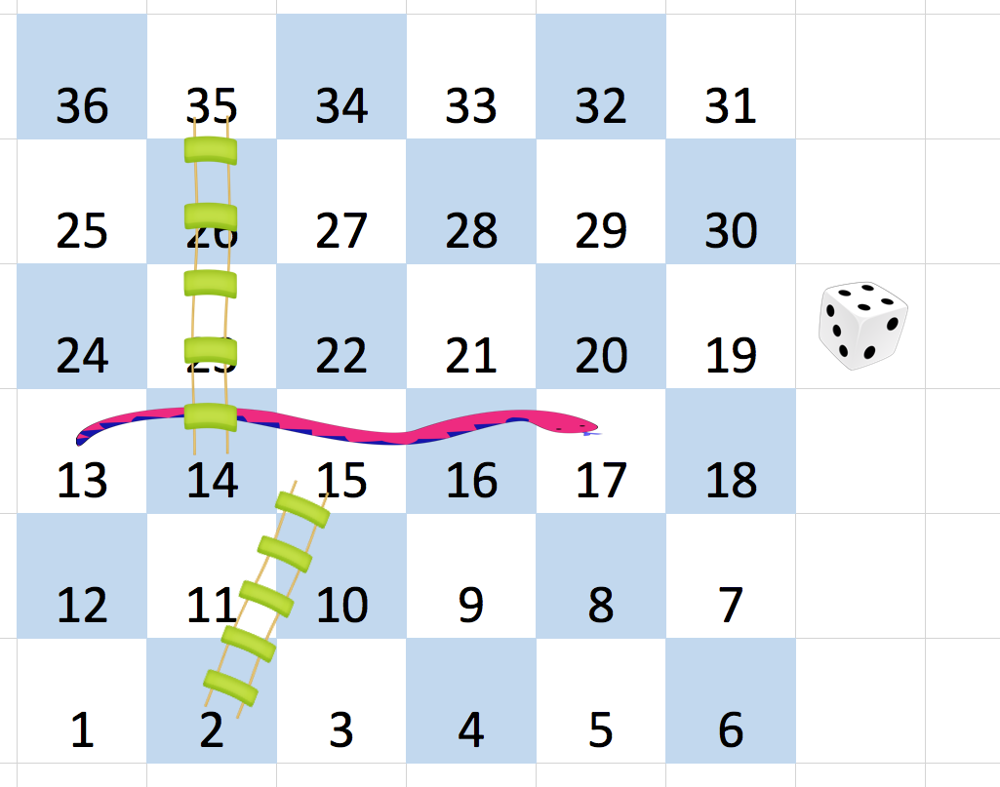On an N x N `board`, the numbers from `1` to `N*N` are written boustrophedonically starting from the bottom left of the board, and alternating direction each row.  For example, for a 6 x 6 board, the numbers are written as follows:

``````

You start on square `1` of the board (which is always in the last row and first column).  Each move, starting from square `x`, consists of the following:

• You choose a destination square `S` with number `x+1`, `x+2`, `x+3`, `x+4`, `x+5`, or `x+6`, provided this number is `<= N*N`.
• (This choice simulates the result of a standard 6-sided die roll: ie., there are always at most 6 destinations, regardless of the size of the board.)
• If `S` has a snake or ladder, you move to the destination of that snake or ladder.  Otherwise, you move to `S`.

A board square on row `r` and column `c` has a "snake or ladder" if `board[r][c] != -1`.  The destination of that snake or ladder is `board[r][c]`.

Note that you only take a snake or ladder at most once per move: if the destination to a snake or ladder is the start of another snake or ladder, you do not continue moving.  (For example, if the board is `[[4,-1],[-1,3]]`, and on the first move your destination square is `2`, then you finish your first move at `3`, because you do not continue moving to `4`.)

Return the least number of moves required to reach square N*N.  If it is not possible, return `-1`.

Example 1:

```Input: [
[-1,-1,-1,-1,-1,-1],
[-1,-1,-1,-1,-1,-1],
[-1,-1,-1,-1,-1,-1],
[-1,35,-1,-1,13,-1],
[-1,-1,-1,-1,-1,-1],
[-1,15,-1,-1,-1,-1]]
Output: 4
Explanation:
At the beginning, you start at square 1 [at row 5, column 0].
You decide to move to square 2, and must take the ladder to square 15.
You then decide to move to square 17 (row 3, column 5), and must take the snake to square 13.
You then decide to move to square 14, and must take the ladder to square 35.
You then decide to move to square 36, ending the game.
It can be shown that you need at least 4 moves to reach the N*N-th square, so the answer is 4.
```

Note:

1. `2 <= board.length = board.length <= 20`
2. `board[i][j]` is between `1` and `N*N` or is equal to `-1`.
3. The board square with number `1` has no snake or ladder.
4. The board square with number `N*N` has no snake or ladder.

``````struct Solution;

use std::collections::VecDeque;

impl Solution {
fn snakes_and_ladders(board: Vec<Vec<i32>>) -> i32 {
let n = board.len();
let mut moves: Vec<i32> = vec![0; n * n];
let mut k = 0;
for i in (0..n).rev() {
if i % 2 != n % 2 {
for j in 0..n {
moves[k] = board[i][j];
k += 1;
}
} else {
for j in (0..n).rev() {
moves[k] = board[i][j];
k += 1;
}
}
}
let mut queue: VecDeque<usize> = VecDeque::new();
let mut visited: Vec<usize> = vec![0; n * n];
visited = 1;
queue.push_back(0);
while let Some(i) = queue.pop_front() {
if i == n * n - 1 {
return visited[i] as i32 - 1;
} else {
for j in i + 1..=i + 6 {
if j >= n * n {
break;
}
let k = if moves[j] == -1 {
j
} else {
(moves[j] - 1) as usize
};
if visited[k] == 0 {
visited[k] = visited[i] + 1;
queue.push_back(k);
}
}
}
}
-1
}
}

#[test]
fn test() {
let board: Vec<Vec<i32>> = [
[-1, -1, -1, -1, -1, -1],
[-1, -1, -1, -1, -1, -1],
[-1, -1, -1, -1, -1, -1],
[-1, 35, -1, -1, 13, -1],
[-1, -1, -1, -1, -1, -1],
[-1, 15, -1, -1, -1, -1],
]
.iter()
.map(|v| v.to_vec())
.collect();
let res = 4;
let board: Vec<Vec<i32>> = [
[-1, -1, 19, 10, -1],
[2, -1, -1, 6, -1],
[-1, 17, -1, 19, -1],
[25, -1, 20, -1, -1],
[-1, -1, -1, -1, 15],
]
.iter()
.map(|v| v.to_vec())
.collect();
let res = 2;